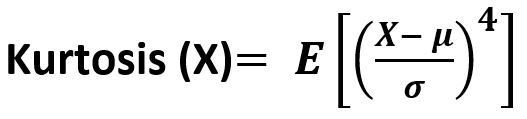GFG App
Open AppBrowser
Continue

# scipy stats.kurtosis() function | Python

scipy.stats.kurtosis(array, axis=0, fisher=True, bias=True) function calculates the kurtosis (Fisher or Pearson) of a data set. It is the fourth central moment divided by the square of the variance. It is a measure of the “tailedness” i.e. descriptor of shape of probability distribution of a real-valued random variable. In simple terms, one can say it is a measure of how heavy tail is compared to a normal distribution. Its formula –Parameters : array : Input array or object having the elements. axis : Axis along which the kurtosis value is to be measured. By default axis = 0. fisher : Bool; Fisher’s definition is used (normal 0.0) if True; else Pearson’s definition is used (normal 3.0) if set to False. bias : Bool; calculations are corrected for statistical bias, if set to False. Returns : Kurtosis value of the normal distribution for the data set.

Code #1:

## Python3

 `# Graph using numpy.linspace() ` `# finding kurtosis`   `from` `scipy.stats ``import` `kurtosis` `import` `numpy as np ` `import` `pylab as p `   `x1 ``=` `np.linspace( ``-``5``, ``5``, ``1000` `)` `y1 ``=` `1.``/``(np.sqrt(``2.``*``np.pi)) ``*` `np.exp( ``-``.``5``*``(x1)``*``*``2`  `)`   `p.plot(x1, y1, ``'*'``)`     `print``( ``'\nKurtosis for normal distribution :'``, kurtosis(y1))`   `print``( ``'\nKurtosis for normal distribution :'``, ` `      ``kurtosis(y1, fisher ``=` `False``))`   `print``( ``'\nKurtosis for normal distribution :'``, ` `      ``kurtosis(y1, fisher ``=` `True``))`

Output :

```
Kurtosis for normal distribution : -0.3073930877422071

Kurtosis for normal distribution : 2.692606912257793

Kurtosis for normal distribution : -0.3073930877422071```
My Personal Notes arrow_drop_up